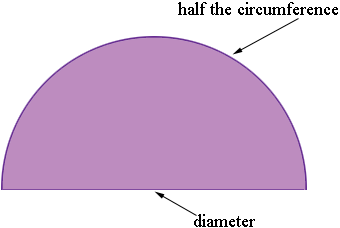SEARCH HOMEMath Central Quandaries & QueriesQuestion from Keith, a parent: I am trying to help my daughter I have the perimeter of a semi circle of 37 but how do I calculate the diameter from this. This is all the information I have. HelpHi Keith,

The perimeter of a semicircle is made up of two parts, a diameter and a curved piece which has the length of half the circumference of the circle.Suppose the diameter is $d$ units long then you know that the circumference of the circle is $\pi d$ units. Thus the perimeter of the semicircle is $d + \frac{\pi}{2} d$ units. But you know that the perimeter is 37 units so

$d + \frac{\pi}{2} d = 37.$

Solve for $d.$

Write back if you need further assistance,
PennyMath Central is supported by the University of Regina and The Pacific Institute for the Mathematical Sciences.